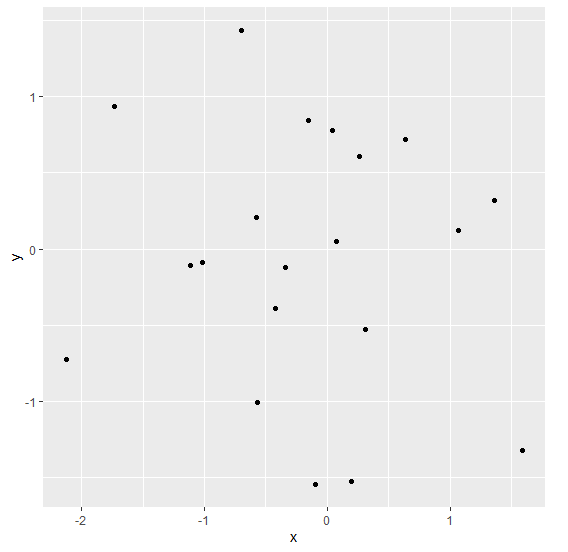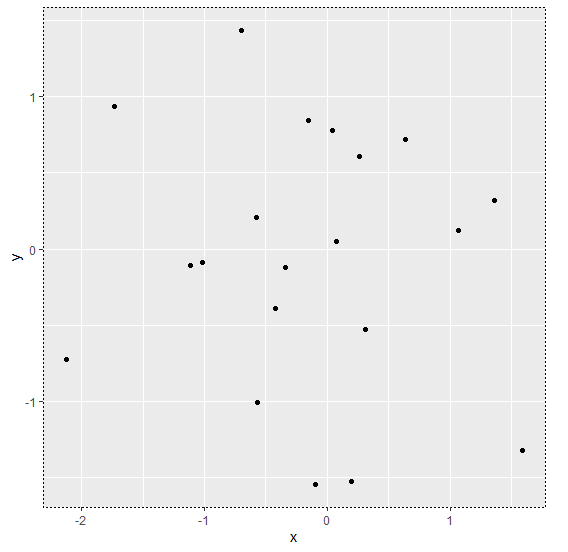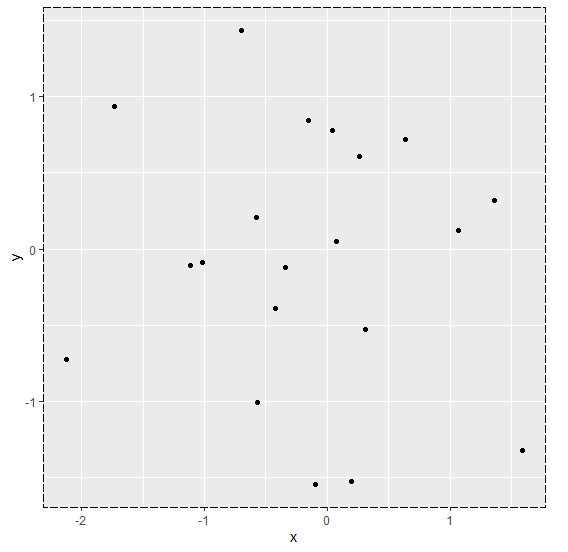# How to change the border style of a plot using ggplot2 in R?

To change the border style of a plot using ggplot2 in R, we can use theme function with panel.border argument where we can change the linetype for the plot border using element_rect. There are many linetypes and we can use them as desired.

Check out the Example given below to understand how it can be done.

## Example

Following snippet creates a sample data frame −

x<-rnorm(20)
y<-rnorm(20)
df<-data.frame(x,y)
df

The following dataframe is created

            x           y
1  0.07659587  0.04662568
2 -0.09346513 -1.54421904
3 -0.15442334  0.84076938
4  0.30773228 -0.52740013
5 -2.12669288 -0.72532478
6  0.04613070  0.77757258
7  0.26543808  0.60828079
8 -1.02039121 -0.08895102
9 -1.73303082  0.93566679
10  1.36236728  0.31907879
11 -0.41927039 -0.38939094
12  1.06971384  0.12146098
13  0.63973606  0.71795862
14 -0.34179992 -0.12283619
15 -1.11315207 -0.10712006
16  0.19498220 -1.52575895
17 -0.58071405  0.20655197
18 -0.70292599  1.43570793
19 -0.57065356 -1.00393263
20  1.59348973 -1.31773976

To load ggplot2 package and create scatterplot between x and y on the above created data frame, add the following code to the above snippet −

x<-rnorm(20)
y<-rnorm(20)
df<-data.frame(x,y)
library(ggplot2)
ggplot(df,aes(x,y))+geom_point()

## Output

If you execute all the above given snippets as a single program, it generates the following Output −To create scatterplot between x and y with different border style on the above created data frame, add the following code to the above snippet −

x<-rnorm(20)
y<-rnorm(20)
df<-data.frame(x,y)
library(ggplot2)
ggplot(df,aes(x,y))+geom_point()+theme(panel.border=element_rect(linetype=3,fill=NA))

## Output

If you execute all the above given snippets as a single program, it generates the following Output −Add the following code to the above snippet −

x<-rnorm(20)
y<-rnorm(20)
df<-data.frame(x,y)
library(ggplot2)
ggplot(df,aes(x,y))+geom_point()+theme(panel.border=element_rect(linetype=11,fill=NA))

## Output

If you execute all the above given snippets as a single program, it generates the following Output −